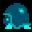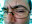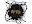# Erik McClure

#### Mathematical Notation Is Awful

Today, a friend asked me for help figuring out how to calculate the standard deviation over a discrete probability distribution. I pulled up my notes from college and was able to correctly calculate the standard deviation they had been unable to derive after hours upon hours of searching the internet and trying to piece together poor explanations from questionable sources. The crux of the problem was, as I had suspected, the astonishingly bad notation involved with this particular calculation. You see, the expected value of a given distribution $$X$$ is expressed as $$E[X]$$, which is calculated using the following formula:

$E[X] = \sum_{i=1}^{\infty} x_i p(x_i)$
The standard deviation is the square root of the variance, and the variance is given in terms of the expected value.
$Var(X) = E[X^2] - (E[X])^2$
Except that $$E[X^2]$$ is of course completely different from $$(E[X])^2$$, but it gets worse, because $$E[X^2]$$ makes no notational sense whatsoever. In any other function, in math, doing $$f(x^2)$$ means going through and substitution $$x$$ with $$x^2$$. In this case, however, $$E[X]$$ actually doesn't have anything to do with the resulting equation, because $$X \neq x_i$$, and as a result, the equation for $$E[X^2]$$ is this:
$E[X^2] = \sum_i x_i^2 p(x_i)$
Only the first $$x_i$$ is squared. $$p(x_i)$$ isn't, because it doesn't make any sense in the first place. It should really be just $$P_{Xi}$$ or something, because it's a discrete value, not a function! It would also explain why the $$x_i$$ inside $$p()$$ isn't squared - because it doesn't even exist, it's just a gross abuse of notation. This situation is so bloody confusing I even explicitely laid out the equation for $$E[X^2]$$ in my own notes, presumably to prevent me from trying to figure out what the hell was going on in the middle of my final.

That, however, was only the beginning. Another question required them to find the covariance between two seperate discrete distributions, $$X$$ and $$Y$$. I have never actually done covariance, so my notes were of no help here, and I was forced to return to wikipedia, which gives this helpful equation.

$cov(X,Y) = E[XY] - E[X]E[Y]$
Oh shit. I've already established that $$E[X^2]$$ is impossible to determine because the notation doesn't rely on any obvious rules, which means that $$E[XY]$$ could evaluate to god knows what. Luckily, wikipedia has an alternative calculation method:
$cov(X,Y) = \frac{1}{n}\sum_{i=1}^{n} (x_i - E(X))(y_i - E(Y))$
This almost works, except for two problems. One, $$\frac{1}{n}$$ doesn't actually work because we have a nonuniform discrete probability distribution, so we have to substitute multiplying in the probability mass function $$p(x_i,y_i)$$ instead. Two, wikipedia refers to $$E(X)$$ and $$E(Y)$$ as the means, not the expected value. This gets even more confusing because, at the beginning of the Wikipedia article, it used brackets ($$E[X]$$), and now it's using parenthesis ($$E(X)$$). Is that the same value? Is it something completely different? Calling it the mean would be confusing because the average of a given data set isn't necessarily the same as finding what the average expected value of a probability distribution is, which is why we call it the expected value. But naturally, I quickly discovered that yes, the mean and the average and the expected value are all exactly the same thing! Also, I still don't know why Wikipedia suddenly switched to $$E(X)$$ instead of $$E[X]$$ because it stills means the exact same goddamn thing.

We're up to, what, five different ways of saying the same thing? At least, I'm assuming it's the same thing, but there could be some incredibly subtle distinction between the two that nobody ever explains anywhere except in some academic paper locked up behind a paywall that was published 30 years ago, because apparently mathematicians are okay with this.

Even then, this is just one instance where the ambiguity and redundancy in our mathematical notation has caused enormous confusion. I find it particularly telling that the most difficult part about figuring out any mathematical equation for me is usually to simply figure out what all the goddamn notation even means, because usually most of it isn't explained at all. Do you know how many ways we have of taking the derivative of something?

$$f'(x)$$ is the same as $$\frac{dy}{dx}$$ or $$\frac{df}{dx}$$ even $$\frac{d}{dx}f(x)$$ which is the same as $$\dot x$$ which is the same as $$Df$$ which is technically the same as $$D_xf(x)$$ and also $$D_xy$$ which is also the same as $$f_x(x)$$ provided x is the only variable, because taking the partial derivative of a function with only one variable is the exact same as taking the derivative in the first place, and I've actually seen math papers abuse this fact instead of use some other sane notation for the derivative. And that's just for the derivative!

Don't even get me started on multiplication, where we use $$2 \times 2$$ in elementary school, $$*$$ on computers, but use $$\cdot$$ or simply stick two things next to each other in traditional mathematics. Not only is using $$\times$$ confusing as a multiplicative operator when you have $$x$$ floating around, but it's a real operator! It means cross product in vector analysis. Of course, the $$\cdot$$ also doubles as meaning the Dot Product, which is at least nominally acceptable since a dot product does reduce to a simple multiplication of scalar values. The Outer Product is generally given as $$\otimes$$, unless you're in Geometric Algebra, in which case it's given by $$\wedge$$, which of course means AND in binary logic. Geometric Algebra then re-uses the cross product symbol $$\times$$ to instead mean commutator product, and also defines the regressive product as the dual of the outer product, which uses $$\nabla$$. This conflicts with the gradient operator in multivariable calculus, which uses the exact same symbol in a totally different context, and just for fun it also defined $$*$$ as the “scalar” product, just to make sure every possible operator has been violently hijacked to mean something completely unexpected.

This is just one area of mathematics - it is common for many different subfields of math to redefine operators into their own meaning and god forbid any of these fields actually come into contact with each other because then no one knows what the hell is going on. Math is a language that is about as consistent as English, and that's on a good day.

I am sick and tired of people complaining that nobody likes math when they refuse to admit that mathematical notation sucks, and is a major roadblock for many students. It is useful only for advanced mathematics that take place in university graduate programs and research laboratories. It's hard enough to teach people calculus, let alone expose them to something useful like statistical analysis or matrix algebra that is relevant in our modern world when the notation looks like Greek and makes about as much sense as the English pronunciation rules. We simply cannot introduce people to advanced math by writing a bunch of incoherent equations on a whiteboard. We need to find a way to separate the underlying mathematical concepts from the arcane scribbles we force students to deal with.

Personally, I understand most of higher math by reformulating it in terms of lambda calculus and type theory, because they map to real world programs I can write and investigate and explore. Interpreting mathematical concepts in terms of computer programs is just one way to make math more tangible. There must be other ways we can explain math without having to explain the extraordinarily dense, outdated notation that we use.Wade Mealing

I end up understanding math by re-writing it in code. it seems to be the only way that I can finally understand how its supposed to work. The only issue is that of course it fails with numbers like infinity, however I still do my best to plod along with some kind of actual implementable logic.John Connors

Mathemeticians should give up on algebraic notation as a mess; instead go with prefix s-expressions the way our new AI overlords will eventually (sensibly) insist upon. :-) I do wonder if we are making a mistake as fundamental as the Roman-conditioned Europe ignoring Arabic numerals.Eustáquio RangelUnknown

Well, the good thing is that people define their notation in papers, due to overlap. The different styles in some sense prepare you to, eh, different styles. Finance on the other hand, is witchcraft - all letters have been defined to mean exactly the same thing all the time, and you're just supposed to know what they mean. Then they invented a bunch of more letters, that sound greek, just for fun, but then, since it is hard to typeset new letters, they use existing greek letters... yeah, f*ck finance..pftq

Funny story on my end is I learned more calculus from programming games than I did in class. I literally averaged Ds, Fs in my first semester before the teacher stopped requiring "proper" work and then it was As from there because my answer was never wrong, just my notation/proofs.Carlos Manzanedo

Absolutely with you on this; Lately I'm finding myself thinking how cool it will be if scientific papers (specially discrete maths) will look more like jupyter notebooks. This is not necessarily the best example but I was quite excited to with how the ligo observations on gravitational waves were explained in this format https://losc.ligo.org/s/events/GW150914/GW150914_tutorial.htmlUnknown

Looks like your course notes are bad. That expression for expected value doesn't make sense, a quick google search shows a wikipedia article with the correct definition: https://en.wikipedia.org/wiki/Expected_value#Univariate_discrete_random_variable.2C_countable_caseErik McClure

My statistics class did not give that definition, it was provided as a function in all cases, consistently, despite being a discrete value. Perhaps I had a terrible statistics teacher?karhunen-loeve

Hi there! I just read your post and wanted to help; I'm a PhD student in pure math so I can really relate to your struggles. Before I start, I'd like to just say that all the equations you pulled from Wikipedia are in fact correct, all this stuff does make sense, and I hope to convince you below that the notation, once understood, is actually very efficient and precise. I would guess that your confusions mostly stem from poor instruction (e.g. professors placing too much emphasis on computations rather than defining things carefully). I'll just start from the top and work my way down, and then you can ask me if anything I say is unclear.

Alright, so at the top you're discussing X, which you refer to as a "distribution", but really, it is an object called a (discrete) random variable. Many courses will gloss over what exactly is meant by a random variable, and say something vague, like "it's just some random numerical quantity". However, as the existence of your post shows, this can easily lead one into a swamp of confusion. So, here's the straight dope: a random variable is really a (measurable) *function*, X, from a *probability space* (what's this? see below), call it Ω, to the set of real numbers. Ignoring the "measurable" part (which is just a technical assumption), this means that X is a thingy which eats sample points (in other words, elements of the probability space Ω aka sample space), and spits out real numbers. For example, consider rolling two dice. The sample space here would be the set {(1,1),(1,2),(1,3),(1,4),(1,5),(1,6),(2,1),(2,2),...,(6,5),(6,6)}, which of course has 6*6=36 elements. An example of a discrete random variable would be the function X that sends a sample point to the sum of the two values, so for example X((6,5)) would just be 6+5 = 11. In a book you would see them write "Define a random variable X to be the sum of the two numbers rolled on the dice", or something. It's important to note that this is sort of misleading, because they're really defining X to be the sum NOT of two numbers, but of two random variables! (Which ones are they?) So there, now you know exactly what the X you're talking about above actually *is*.

Above I should have also told you what "probability space" means. Well, it's basically a set, equipped with a "probability measure": a way of assigning probabilities (numbers between 0 and 1) to certain subsets which people call "events". Why only *certain* subsets you ask? Uhhh... this is a technicality and not really relevant to understanding things for now. It's because by invoking the Axiom of Choice and constructing some awful monsters, you can show that in a lot of natural scenarios you can't find a consistent notion of measure that works for *all* subsets. You can read more about this in any introductory treatment of the Lebesgue measure.karhunen-loeve

Moving on, you say that "E[X]" (which, yes, is the same thing as "E(X)" -- mathematics is done by humans after all, and for better or worse there will always be such minor variations among people's notational preferences) is bad notation. Actually, it's fine though: I mean, at the end of the day it *is* just a notation and nothing more, but you can roughly think about E[-] as being some kind of function that consumes *random variables*, and produces a real number as output (called the "expectation", "expected value", or, indeed, also the "mean" of the r.v.). Thus, even though X does not "equal x_i", as you say, those numbers p(x_i) *are* part of the data packaged into X. [How? Well, the domain Ω of X is a probability space, which means it comes equipped with a gadget called a "probability measure", P, that allows us to decide how "large" subsets of Ω are. Thus, p(x_i) just means P({w in Ω such that X(w) = x_i}), or in words, it's the probability that an outcome occurs to which X assigns the numerical value x_i. Remember what I said above about X being a function from Ω to the reals.]

OK, next you say E[X^2] isn't actually equal to (E[X])^2. This is true, and it's good, because otherwise the variance would always give zero! To understand what the notation "E[X^2]" means, note that X^2 is a *new* random variable. Rigorously (going back to our definition of random variables as functions), it is the *composition* of the function X : Ω->R with the squaring map g : R->R, that is, X^2 = g circle X, as a function. Intuitively, you can think of X^2 like this: to get the probability that X^2=x for some x, you basically just find the probabilities that X=+sqrt(x) and X=-sqrt(x), and add 'em together. Pretty natural, right? The same reasoning applies to XY: it is a *new* discrete random variable, and to find out the probability that, say, XY=10, you have to say "hmm, well if X and Y both take on positive integer values, how could we possibly have XY=10? well, we could have X=1 and Y=10, or X=2 and Y=5, or X=5 and Y=2, or X=10 and Y=1"... and then add up all those probabilities! (Note that saying things like "X=1" is teeechnically abusive, because X is NOT a number -- it's a random variable, i.e. one of these "measurable functions", and functions can never equal numbers; it's a type mismatch -- but people do this all the time because it's more intuitive to think of these as "realizations" of a random variable than the rigorous way, which would be writing the awful eyesore P({w in Ω : X(w)Y(w) = 10}) in place of Pr(XY=10).)

Again, hope I didn't just confuse you with all this. Please let me know if anything is unclear, I'd be glad to help more.karhunen-loeve

The second half of your post is something I'd like to discuss a little more. To start I'll just say that (regarding derivatives for example), the "df/dx versus f'(x)" thing essentially goes back to Leibniz vs Newton, while the other notations arose for various reasons: the "dotted-variable" notation is ONLY used to denote differentiation with respect to time (because this operation is just *so* common, e.g. in classical mechanics but really physics in general), while the other notations (Jacobian matrices, gradients, etc.) were likely invented to emphasize that multivariable functions were at play. To understand this, it usually suffices to study the relevant areas for a while. For example, if you learn some introductory Lagrangian mechanics you'll see just how useful it is to be able to write dots over certain things...

If you look in old physics/engineering books you will see all sorts of weird stuff: people putting arrows over vectors, or typesetting them in bold face, etc. It also appears in lower-division math classes, likely because it's expected to lessen the cognitive load on novice students, specifically, by removing or at least diminishing their need to remember the *types* of the objects they're manipulating. However, the overwhelming majority of modern math books, at least pure math books, don't do this. They will write "x" without even batting an eye, whether x is a number, or a vector in R^n, a point on a manifold, or an element of some crazy Fréchet space or something. The expectation is that, for example, if I say "Suppose x is in R^n", then technically you *have* been told that it's a vector, by virtue of it being an element of R^n, and you should not need to be reminded. This ties into the later part of your post: it is very true that mathematics has sprawled into an enormous tree. It's quite common, unfortunately, for two branches (even nearby branches!) to be mutually unintelligible. But I've also grown to accept the present state of affairs since I genuinely believe that striving for absolute uniqueness in notation, as you seem to suggest, not only has diminishing returns, but is just straight up *impossible*. What are your thoughts?